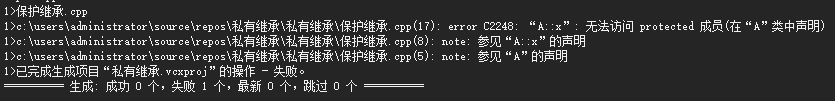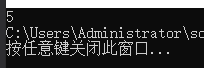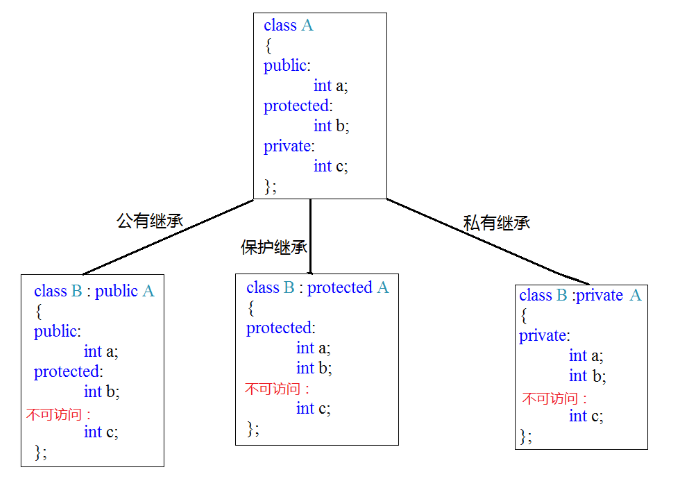# c++继承方式

派生类继承了基类中除了构造和析构函数外所有部分，并且基类成员在子类中访问属性取决于继承方式。c++类中的成员函数和变量有三种继承方式，分别为：公有继承（public），私有继承（private）和保护继承（protect）。

public:用该关键字修饰的成员表示公有成员，该成员不仅可以在类内可以被访问，在类外也是可以被访问的，是类对外提供的可访问接口；
private:用该关键字修饰的成员表示私有成员，该成员仅在类内可以被访问，在类体外是隐藏状态；

protected:用该关键字修饰的成员表示保护成员，保护成员在类体外同样是隐藏状态，但是对于该类的派生类来说，相当于公有成员，在派生类中可以被访问。

公有继承时，基类的公有成员和保护成员在派生类中属性不变，但私有成员不可直接访问。基类成员对派生类对象的可见性为：基类的公有成员可见，保护成员和私有成员不可见，即通过派生类的对象只能访问基类的public成员。
所以，在公有继承时，派生类的对象可以直接访问基类中的公有成员，派生类的成员函数可以直接访问基类中的公有成员和保护成员。

#include<iostream>

using namespace std;

class point{

private: int x, y;

public:

void initpoint(float x1 = 0, float y1 = 0) {

x = x1;
y = y1;
}

void move(float xx=0, float yy=0) {

x += xx;
y += yy;

}

float getx() const{
return x;
}

float gety() const {
return y;
}

};

class Rectangle :public point {   //公有继承

private:

int w, h;

public:
void initRectangle(float x, float y, float w, float h) {

initpoint(x, y);

this->w = w;
this->h = h;
}

float geth() const {
return h;
}

float getw() const {
return w;

}
};

int main() {

Rectangle rect;

rect.initRectangle(2, 3, 20, 30);

rect.move(3, 2);

cout << rect.getx() << endl;
cout << rect.gety() << endl;
cout << rect.getw() << endl;
cout << rect.geth() << endl;

return 0;
}我们可以看出，在初始化时，由于不能直接初始化基类私有成员，我们直接调用了initpoint这个函数接口。这个函数是基类中的，由于是public方式直接可以使用此基类成员函数。

当继承方式为私有继承时，基类的公有成员和保护成员都以私有成员出现在派生类中，同样私有成员在派生类中不可直接访问。此时派生类成员函数可以调用基类公有成员和保护成员，但是在类外部不可使用任何基类成员。可以看出，再进一步派生的话，基类所有成员就无法被直接访问。一般私有继承的使用比较少。

#include<iostream>

using namespace std;

class point {

private: int x, y;

public:

void initpoint(float x1 = 0, float y1 = 0) {

x = x1;
y = y1;
}

void move(float xx = 0, float yy = 0) {

x += xx;
y += yy;

}

float getx() const {
return x;
}

float gety() const {
return y;
}

};

class Rectangle :private point {   //私有继承

private:

int w, h;

public:
void initRectangle(float x, float y, float w, float h) {

initpoint(x, y);

this->w = w;
this->h = h;
}

float getx() const {
return point::getx();
}

float gety() const {
return point::gety();
}

float geth() const {
return h;
}

float getw() const {
return w;

}

void move(float offx, float offy) {

point::move(offx, offy);
}
};

int main() {

Rectangle rect;

rect.initRectangle(2, 3, 20, 30);

rect.move(1, 1);

cout << rect.getx() << endl;
cout << rect.gety() << endl;
cout << rect.getw() << endl;
cout << rect.geth() << endl;

return 0;
}需要注意的是，私有继承后基类成员函数只能在派生类函数中使用，在外部引用会报错。如派生类直接调用move函数时就会报错。为了保证派生类继续拥有基类的接口，就需要重新声明相同的成员例如上例重新声明了getx()，gety()，和move()函数，根据基类隐藏原则基类函数被隐藏，但实现的是相同的效果。

在保护继承中，基类公有成员和保护成员都以保护成员出现在派生类中，但基类私有成员仍然不能被直接访问。保护在派生类中被视为公有成员，在类外被视为私有成员。他结合了公有成员和私有成员的优点，更合理地保护成员。私有继承和保护继承的区别中。所有成员的访问属性都完全相同，但是如果派生类继续派生，保护继承下的基类成员一直都存在派生类基类中，但是私有成员将无法访问基类成员。
保护继承既实现成员隐蔽，又方便继承。

来看一个错误例子。

#include<iostream>

using namespace std;

class A {

protected:

int x;

};

int main() {

A a;

a.x = 1;

return 0;
}


我们看出他会报错。在没有派生类的情况下，保护类型和私有类型相似，他们都不能在类外访问，否则会报错。但是如果A类以公有派生产生了B类，则在B类中，A中的保护成员像公有成员一样可以直接调用。

#include<iostream>

using namespace std;

class A {

protected:
int x;

};

class B:public A{

public:

void fuc(){

x = 5;

cout << x;
}

};

int main() {

B b;

b.fuc();

return 0;
}需要注意的是，如果B是A的派生，B中的成员函数只能通过B的对象访问A中定义的protected成员，而不能通过A的对象访问A中的protected成员。

三中继承方式总结为下图：posted on 2019-10-13 22:06  夏千意  阅读(2918)  评论(0编辑  收藏  举报